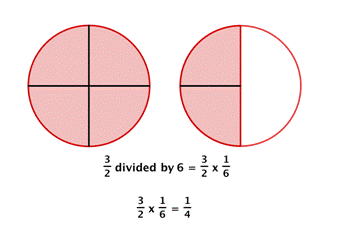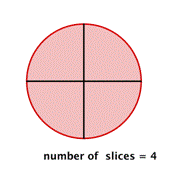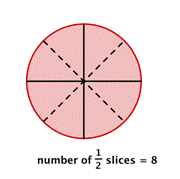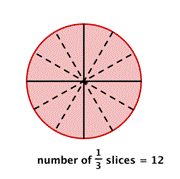Dividing Fractions

Learning Outcomes

• Divide fractions
• Find the reciprocal of a number
• Divide a fraction by a whole number
• Divide a fraction by a fraction

Introduction

Before we get started, here is some important terminology that will help you understand the concepts about working with fractions in this section.

• product: the result of  multiplication
• factor: something being multiplied – for  $3 \cdot 2 = 6$ , both 3 and 2 are factors of 6
• numerator: the top part of a fraction – the numerator in the fraction $\frac{2}{3}$ is 2
• denominator: the bottom part of a fraction – the denominator in the fraction $\frac{2}{3}$ is 3

Many different words are used by math textbooks and teachers to provide students with instructions on what they are to do with a given problem. For example, you may see instructions such as “Find” or “Simplify” in the example in this module. It is important to understand what these words mean so you can successfully work through the problems in this course. Here is a short list of the words you may see that can help you know how to work through the problems in this module.

Instruction Interpretation
Find Perform the indicated mathematical operations which may include addition, subtraction, multiplication, division.
Simplify 1) Perform the indicated mathematical operations including addition, subtraction, multiplication, division

2) Write a mathematical statement in smallest terms so there are no other mathematical operations that can be performed—often found in problems related to fractions and the order of operations

Evaluate Perform the indicated mathematical operations including addition, subtraction, multiplication, division
Reduce Write a mathematical statement in smallest or lowest terms so there are no other mathematical operations that can be performed—often found in problems related to fractions or division

Divide Fractions

There are times when you need to use division to solve a problem. For example, if painting one coat of paint on the walls of a room requires 3 quarts of paint and you have a bucket that contains 6 quarts of paint, how many coats of paint can you paint on the walls? You divide 6 by 3 for an answer of 2 coats. There will also be times when you need to divide by a fraction. Suppose painting a closet with one coat only required $\frac{1}{2}$ quart of paint. How many coats could be painted with the 6 quarts of paint? To find the answer, you need to divide 6 by the fraction, $\frac{1}{2}$.

Before we begin dividing fractions, let’s cover some important terminology.

• reciprocal: two fractions are reciprocals if their product is 1 (Don’t worry; we will show you examples of what this means.)
• quotient: the result of division

Dividing fractions requires using the reciprocal of a number or fraction. If you multiply two numbers together and get 1 as a result, then the two numbers are reciprocals. Here are some examples of reciprocals:

Original number Reciprocal Product
$\frac{3}{4}$ $\frac{4}{3}$ $\frac{3}{4}\cdot \frac{4}{3}=\frac{3\cdot 4}{4\cdot 3}=\frac{12}{12}=1$
$\frac{1}{2}$ $\frac{2}{1}$ $\frac{1}{2}\cdot\frac{2}{1}=\frac{1\cdot}{2\cdot1}=\frac{2}{2}=1$
$3=\frac{3}{1}$ $\frac{1}{3}$ $\frac{3}{1}\cdot \frac{1}{3}=\frac{3\cdot 1}{1\cdot 3}=\frac{3}{3}=1$
$2\frac{1}{3}=\frac{7}{3}$ $\frac{3}{7}$ $\frac{7}{3}\cdot\frac{3}{7}=\frac{7\cdot3}{3\cdot7}=\frac{21}{21}=1$

Sometimes we call the reciprocal the “flip” of the other number: flip $\frac{2}{5}$ to get the reciprocal $\frac{5}{2}$.

Division by Zero

You know what it means to divide by 2 or divide by 10, but what does it mean to divide a quantity by 0? Is this even possible? Can you divide 0 by a number? Consider the fraction

$\frac{0}{8}$

We can read it as, “zero divided by eight.” Since multiplication is the inverse of division, we could rewrite this as a multiplication problem.

$\text{?}\cdot{8}=0$.

We can infer that the unknown must be 0 since that is the only number that will give a result of 0 when it is multiplied by 8.

Now let’s consider the reciprocal of $\frac{0}{8}$ which would be $\frac{8}{0}$. If we rewrite this as a multiplication problem, we will have

$\text{?}\cdot{0}=8$.

This doesn’t make any sense. There are no numbers that you can multiply by zero to get a result of 8. The reciprocal of $\frac{8}{0}$ is undefined, and in fact, all division by zero is undefined.Caution! Division by zero is undefined and so is the reciprocal of any fraction that has a zero in the numerator. For any real number a, $\frac{a}{0}$ is undefined. Additionally, the reciprocal of $\frac{0}{a}$ will always be undefined.

Divide a Fraction by a Whole Number

When you divide by a whole number, you are multiplying by the reciprocal. In the painting example where you need 3 quarts of paint for a coat and have 6 quarts of paint, you can find the total number of coats that can be painted by dividing 6 by 3, $6\div3=2$. You can also multiply 6 by the reciprocal of 3, which is $\frac{1}{3}$, so the multiplication problem becomes

$\frac{6}{1}\cdot \frac{1}{3}=\frac{6}{3}=2$.

Dividing is Multiplying by the Reciprocal

For all division, you can turn the operation into multiplication by using the reciprocal. Dividing is the same as multiplying by the reciprocal.

The same idea will work when the divisor (the thing being divided) is a fraction. If you have $\frac{3}{4}$ of a candy bar and need to divide it among 5 people, each person gets $\frac{1}{5}$ of the available candy:

$\frac{1}{5}\text{ of }\frac{3}{4}=\frac{1}{5}\cdot \frac{3}{4}=\frac{3}{20}$

Each person gets $\frac{3}{20}$ of a whole candy bar.

If you have a recipe that needs to be divided in half, you can divide each ingredient by 2, or you can multiply each ingredient by $\frac{1}{2}$ to find the new amount.

For example, dividing by 6 is the same as multiplying by the reciprocal of 6, which is $\frac{1}{6}$. Look at the diagram of two pizzas below.  How can you divide what is left (the red shaded region) among 6 people fairly?Each person gets one piece, so each person gets $\frac{1}{4}$ of a pizza.

Dividing a fraction by a whole number is the same as multiplying by the reciprocal, so you can always use multiplication of fractions to solve division problems.

Example

Find $\frac{2}{3}\div 4$.

Example

Divide. $9\div\frac{1}{2}$.

Divide a Fraction by a Fraction

Sometimes you need to solve a problem that requires dividing by a fraction. Suppose you have a pizza that is already cut into 4 slices. How many $\frac{1}{2}$ slices are there?There are 8 slices. You can see that dividing 4 by $\frac{1}{2}$ gives the same result as multiplying 4 by 2.

What would happen if you needed to divide each slice into thirds?You would have 12 slices, which is the same as multiplying 4 by 3.

Dividing with Fractions

1. Find the reciprocal of the number that follows the division symbol.
2. Multiply the first number (the one before the division symbol) by the reciprocal of the second number (the one after the division symbol).

Any easy way to remember how to divide fractions is the phrase “keep, change, flip.” This means to KEEP the first number, CHANGE the division sign to multiplication, and then FLIP (use the reciprocal) of the second number.

Example

Divide $\frac{2}{3}\div \frac{1}{6}$.

Example

Divide $\frac{3}{5}\div \frac{2}{3}$.